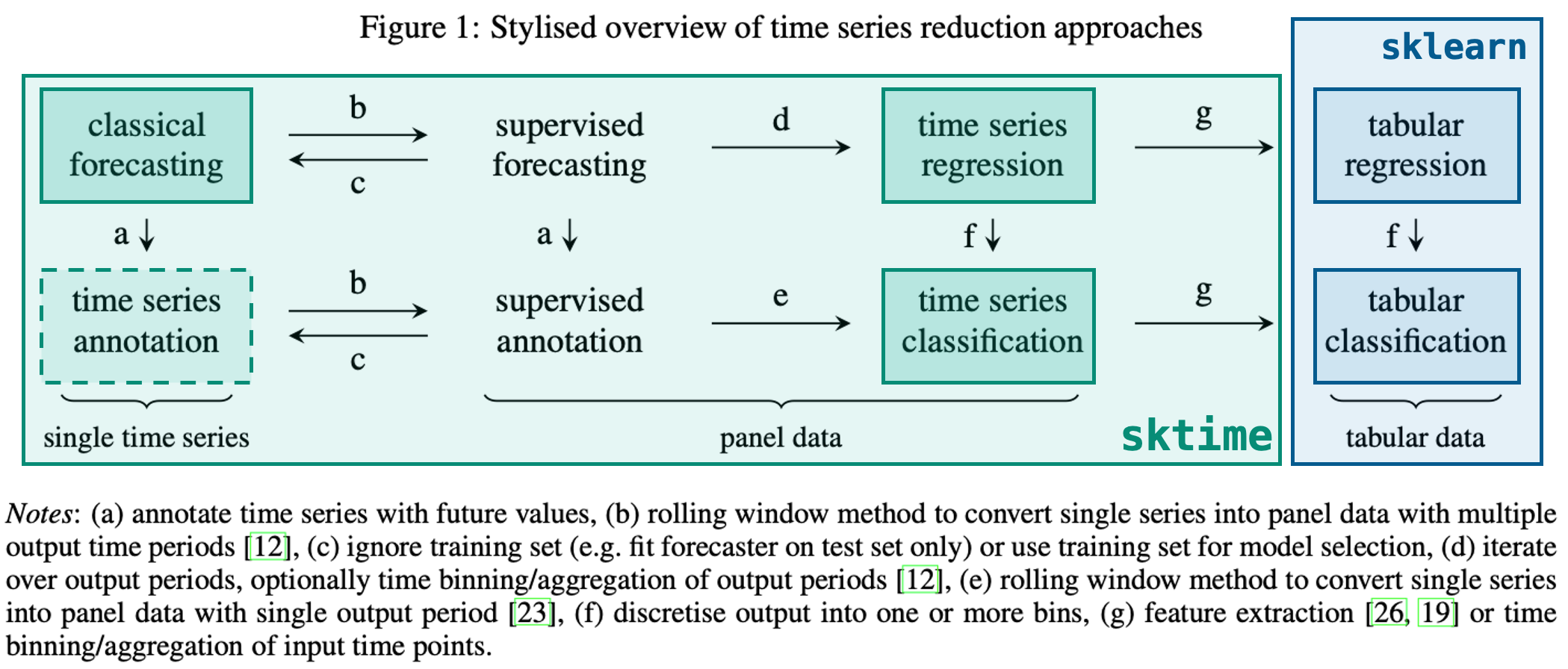# Time Series Forecasting

Created: Time Series Forecasting using SKTime and SKLearn

View this Notebook on Kaggle

# Forecasting with SKTime

SKTime Docs## Resources

# This Python 3 environment comes with many helpful analytics libraries installed
# It is defined by the kaggle/python Docker image: https://github.com/kaggle/docker-python
# Input data files are available in the read-only "../input/" directory
# For example, running this (by clicking run or pressing Shift+Enter) will list all files under the input directory

import os
for dirname, _, filenames in os.walk('/kaggle/input'):
for filename in filenames:
print(os.path.join(dirname, filename))

# You can write up to 20GB to the current directory (/kaggle/working/) that gets preserved as output when you create a version using "Save & Run All"
# You can also write temporary files to /kaggle/temp/, but they won't be saved outside of the current session

!pip install sktime
!pip install pmdarima

import numpy as np
import pandas as pd
import seaborn as sns
import sktime as sktime
import matplotlib.pyplot as plt


The basic workflow when using SKTime is as follows:

1. Specify data
3. Specify model
4. Fit
5. Predict

SKTime also provides some sample datasets and other utilities under the sktime namespace:

from sktime.datasets import load_shampoo_sales
from sktime.utils.plotting import plot_series

df_shampoo = load_shampoo_sales()
plot_series(df_shampoo)

(<Figure size 1152x288 with 1 Axes>,
, <AxesSubplot:ylabel='Number of shampoo sales'>)

<Figure size 1152x288 with 1 Axes>## Forecasting

Forecasting works by taking the input data and trying to calculate what the data will be after X time period

This requires us to define a ForecastingHorizon which is the period of time over which we want to predict. sktime also has helpers for these

from sktime.forecasting.base import ForecastingHorizon

# timeframe to predict from
prediction_start = df_shampoo[-6:].index

prediction_range = pd.period_range(prediction_start.start_time, freq=prediction_start.freqstr, periods=6)

prediction_range

PeriodIndex(['1993-07', '1993-08', '1993-09', '1993-10', '1993-11', '1993-12'], dtype='period[M]')

fh_shampoo = ForecastingHorizon(
prediction_range,
is_relative=False
)

train_cutoff = df_shampoo[-6:].index

train_cutoff

Period('1993-07', 'M')


## Train/Test Split

Splitting train and test data can be done by specifying the forecasting horizon, this will return a test set and train set where the test set is in the forecasting horizon

from sktime.forecasting.model_selection import temporal_train_test_split

y_train, y_test = temporal_train_test_split(df_shampoo, fh=fh_shampoo)

plot_series(y_train, y_test, labels=["y_train", "y_test"])

(<Figure size 1152x288 with 1 Axes>,
, <AxesSubplot:ylabel='Number of shampoo sales'>)

<Figure size 1152x288 with 1 Axes>## Forecasting Based on Test/Train Data

This is done similar to sklearn models:

1. Instantiate model
2. Fit model
3. Predict
4. Evaluate

To enable this methodology, sktime provides different forecasting models that can be used. Below is an example using a NaiveForecaster:

from sktime.forecasting.naive import NaiveForecaster
NaiveForecaster?

forecaster = NaiveForecaster(strategy="drift", window_length=10)
forecaster.fit(y_train)

NaiveForecaster(strategy='drift', window_length=10)


Once fitted, generate predictions using the ForecastingHorizon that was defined for the prediction period

y_pred = forecaster.predict(fh_shampoo)

plot_series(y_train, y_test, y_pred, labels=["y_train", "y_test", "y_pred"])

(<Figure size 1152x288 with 1 Axes>,
, <AxesSubplot:ylabel='Number of shampoo sales'>)

<Figure size 1152x288 with 1 Axes>## Model Evaluation

from sktime.performance_metrics.forecasting import mean_absolute_percentage_error

mean_absolute_percentage_error(y_test, y_pred)

0.16469764622516225


## ARIMA Example

We can also use an ARIMA model for example as follows:

from sktime.forecasting.arima import AutoARIMA

# sp=12 for monthly data seasonality
forecaster = AutoARIMA(sp=12, suppress_warnings=True)
forecaster.fit(y_train)

AutoARIMA(sp=12, suppress_warnings=True)

y_pred = forecaster.predict(fh=fh_shampoo)
plot_series(y_train, y_test, y_pred, labels=["y_train", "y_test", "y_pred"])

(<Figure size 1152x288 with 1 Axes>,
, <AxesSubplot:ylabel='Number of shampoo sales'>)

<Figure size 1152x288 with 1 Axes>## Using SKLearn Regressors

sktime also supports using sklearn regressors and supports transforming them into time-series compatible regressors by way of the make_reduction function:

from sklearn.neighbors import KNeighborsRegressor
from sktime.forecasting.compose import make_reduction


y_train, y_test = temporal_train_test_split(airline_df, test_size=12)
plot_series(y_train, y_test, labels=["y_train", "y_test"])

(<Figure size 1152x288 with 1 Axes>,
, <AxesSubplot:ylabel='Number of airline passengers'>)

<Figure size 1152x288 with 1 Axes>fh = ForecastingHorizon(y_test.index, is_relative=False)


transform a regressor into a forecaster

regressor = KNeighborsRegressor(n_neighbors=3)
forecaster = make_reduction(regressor, strategy="recursive", window_length=12)

forecaster.fit(y_train, fh=fh)

RecursiveTabularRegressionForecaster(estimator=KNeighborsRegressor(n_neighbors=3),
,                                     window_length=12)

y_pred = forecaster.predict(fh=fh)
plot_series(y_train, y_test, y_pred, labels=["y_train", "y_test", "y_pred"])

(<Figure size 1152x288 with 1 Axes>,
, <AxesSubplot:ylabel='Number of airline passengers'>)

<Figure size 1152x288 with 1 Axes>